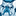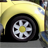Announcements
Sept. 27, 10AM ET Do more with Qlik, Insight Advisor – our intelligent AI-assistant in Qlik Sense: Register
cancel
Showing results for
Did you mean:Not applicable

## Fomula issue with set analysis

Hi all,

I am having problems with this formula, which I want to extend to the rest of the months:

((sum({\$<Escenario={ \$(=Only(Esc_1))},Month = {'Jan'},Channel = {'CallCenter'} >} Rental_Days * (1+[vRDs_Av_CALLCENTER_1])))

/

((sum({\$<Escenario={ \$(=Only(Esc_1))},Month = {'Jan'},Channel = {'CallCenter'} >} Rental_Days * (1+[vRA_Av_CALLCENTER_1])))

/

(sum({\$<Escenario={ \$(=Only(Esc_1))},Month = {'Jan'},Channel = {'CallCenter'} >} Contador_RAs))))

+

((sum({\$<Escenario={ \$(=Only(Esc_1))},Month = {'Feb'},Channel = {'CallCenter'} >} Rental_Days * (1+[vRDs_Av_CALLCENTER_2])))

/

((sum({\$<Escenario={ \$(=Only(Esc_1))},Month = {'Feb'},Channel = {'CallCenter'} >} Rental_Days * (1+[vRA_Av_CALLCENTER_2])))

/

(sum({\$<Escenario={ \$(=Only(Esc_1))},Month = {'Feb'},Channel = {'CallCenter'} >} Contador_RAs))))

Just letting this formula like this, if I select the two months (Jan and Feb), I got the right number. However, if I select just one month, the value doesn´t apper. I guess because of the one I dont select result in null, also because of the divide in the formula.

Could anyone help me to try to convert the null in a 0 or something like that?

Many thanks!

Antonio.

1 Solution

Accepted SolutionsMaster II

Can you please try replacing the SUM with NUMSUM and see if it helps..

6 RepliesSpecialist

Use if statement

((sum({\$<Escenario={ \$(=Only(Esc_1))},Month = {'Jan'},Channel = {'CallCenter'} >} Rental_Days * (1+[vRDs_Av_CALLCENTER_1])))

/

((sum({\$<Escenario={ \$(=Only(Esc_1))},Month = {'Jan'},Channel = {'CallCenter'} >} Rental_Days * (1+[vRA_Av_CALLCENTER_1])))

/

(sum({\$<Escenario={ \$(=Only(Esc_1))},Month = {'Jan'},Channel = {'CallCenter'} >} Contador_RAs))))

))<1,0,

((sum({\$<Escenario={ \$(=Only(Esc_1))},Month = {'Jan'},Channel = {'CallCenter'} >} Rental_Days * (1+[vRDs_Av_CALLCENTER_1])))

/

((sum({\$<Escenario={ \$(=Only(Esc_1))},Month = {'Jan'},Channel = {'CallCenter'} >} Rental_Days * (1+[vRA_Av_CALLCENTER_1])))

/

(sum({\$<Escenario={ \$(=Only(Esc_1))},Month = {'Jan'},Channel = {'CallCenter'} >} Contador_RAs))))

)

)Not applicable
Author

Hi Rupam,

Thanks for your response. However, it doesnt work.

Do you have any other idea??

Many thanks!!Not applicable
Author

Hi,

I would really appreciate it.

Many thanks!!Master II

Can you please try replacing the SUM with NUMSUM and see if it helps..Not applicable
Author

Ey!

It works!

Many thanksAtonio.Master II

You are welcome...Community Browser